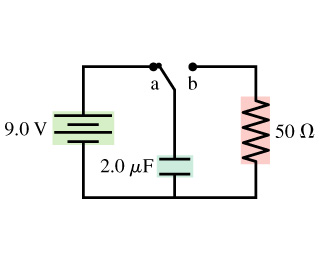# Problem: The switch in the figure (Figure 1) has been in position a for a long time. It is changed to position b at t=0s.Part AWhat is the charge Q on the capacitor immediately after the switch is moved to position b? Express your answer using two significant figures.Q = ______  CPart BWhat is the current I through the resistor immediately after the switch is moved to position b? Express your answer using two significant figures.I = ______ mAPart CWhat is the charge Q on the capacitor at t=50 μs? Express your answer using two significant figures.Q = ______ CPart DWhat is the current I through the resistor at t=50μs? Express your answer using two significant figures.I = ______ mAPart EWhat is the charge Q on the capacitor at t=200 μs? Express your answer using two significant figures.Q = ______ CPart FWhat is the current I through the resistor at t=200μs? Express your answer using two significant figures.I = ______ mA

###### FREE Expert Solution

Part A

Charge:

$\overline{){\mathbf{Q}}{\mathbf{=}}{\mathbf{C}}{\mathbf{V}}}$

87% (109 ratings)###### Problem Details

The switch in the figure (Figure 1) has been in position a for a long time. It is changed to position b at t=0s.Part A

What is the charge Q on the capacitor immediately after the switch is moved to position b? Express your answer using two significant figures.

Q = ______  C

Part B

What is the current I through the resistor immediately after the switch is moved to position b? Express your answer using two significant figures.

I = ______ mA

Part C

What is the charge Q on the capacitor at t=50 μs? Express your answer using two significant figures.

Q = ______ C

Part D

What is the current I through the resistor at t=50μs? Express your answer using two significant figures.

I = ______ mA

Part E

What is the charge Q on the capacitor at t=200 μs? Express your answer using two significant figures.

Q = ______ C

Part F

What is the current I through the resistor at t=200μs? Express your answer using two significant figures.

I = ______ mA

Frequently Asked Questions

What scientific concept do you need to know in order to solve this problem?

Our tutors have indicated that to solve this problem you will need to apply the !! Resistor-Capacitor Circuits concept. If you need more !! Resistor-Capacitor Circuits practice, you can also practice !! Resistor-Capacitor Circuits practice problems.

What professor is this problem relevant for?

Based on our data, we think this problem is relevant for Professor Ertl's class at UMASS.